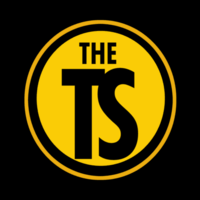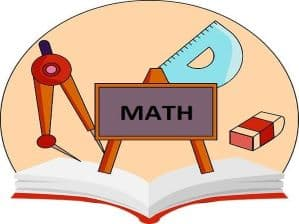# BJC MATHEMATICS (Non-Interactive)\$ 10.00Mathematics is the science of numbers, quantities and spaces which can be used for abstract concepts or can be applied to other subjects, such as engineering and physics. This course will prepare students to take Paper 1 and Paper 2 of the Mathematics BJC. The BJC aims to test candidates’ ability to apply and utilize mathematical concepts in a variety of areas such as everyday problem solving and analysis of data. Students will learn basic elements for solving problems. They will also develop an understanding of techniques used to solve these problems in preparation for higher level mathematical examinations.

COURSE OBJECTIVES:

–Develop mathematical knowledge and skills with emphasis on confidence and satisfaction.

–To understand problem solving techniques used to solve equations and word problems.

–Develop a feel for performing operations and demonstrating the importance of results found.

–To recognize how a situation can be represented mathematically and identifying the relevant factors used to arrive at a solution.

–Develop the ability to generalize, prove and classify different aspects of mathematical expressions.

–To produce an appreciation for patterns and the relationships between mathematical concepts.

–To acquire a foundation appropriate for further study of mathematics and its branch disciplines.

HERE IS THE SYLLABUS THAT CORRESPONDS TO THE LESSONS BELOW

MATH BJC SYLLABUS

### Course Features

• Lectures 9
• Quizzes 0
• Students 20
• Assessments Yes

The Tutoring Station is an interactive online learning platform that seeks to address the educational needs of a wide variety of traditional and nontraditional students. We offer online courses on a range of subjects as we aim to affect academic achievement and personal and professional development. We also seek to create better working opportunities for educators.